Successfully reported this slideshow.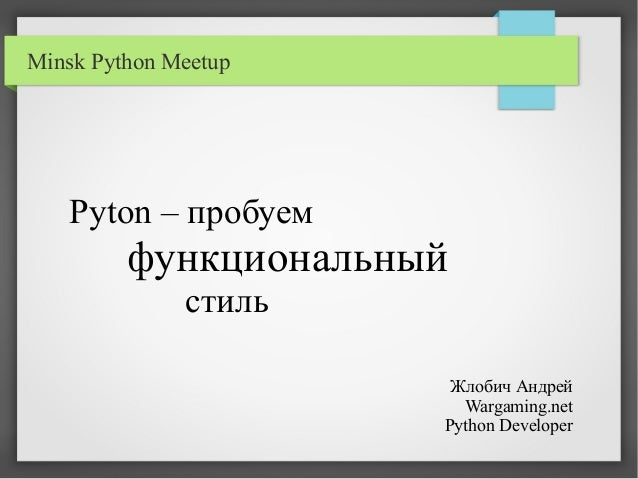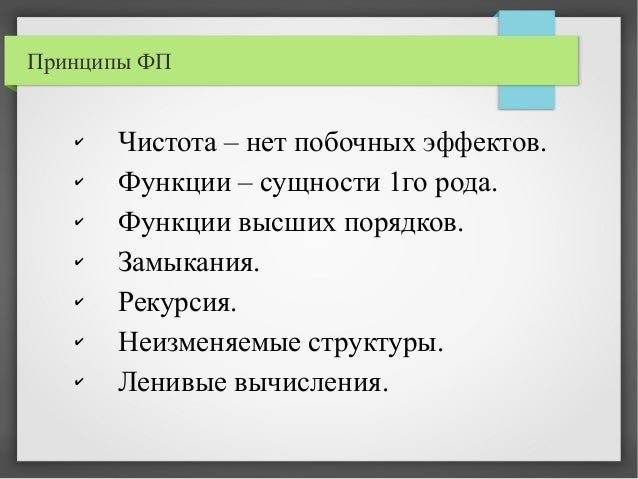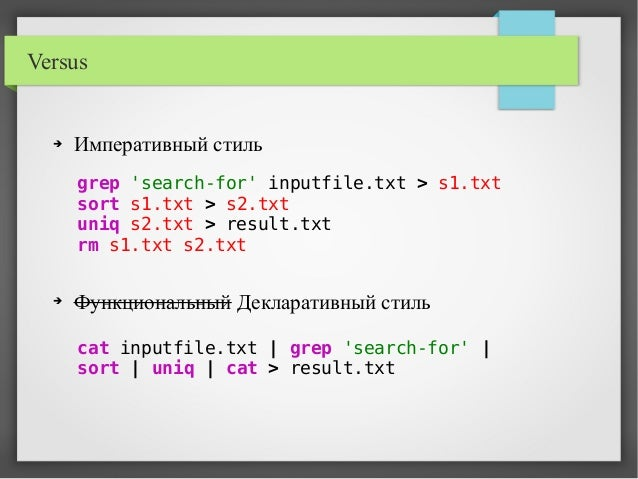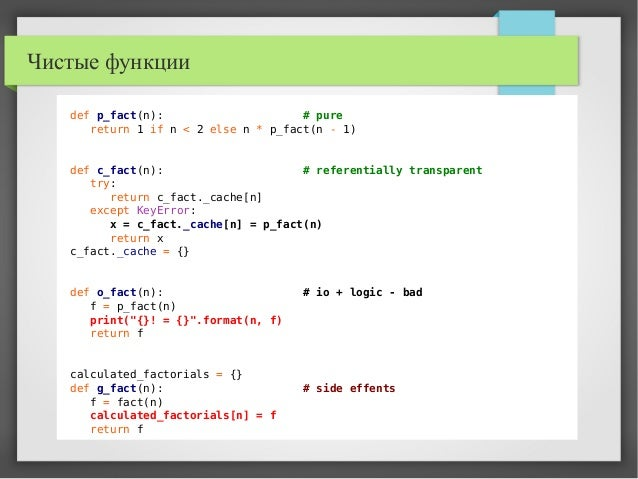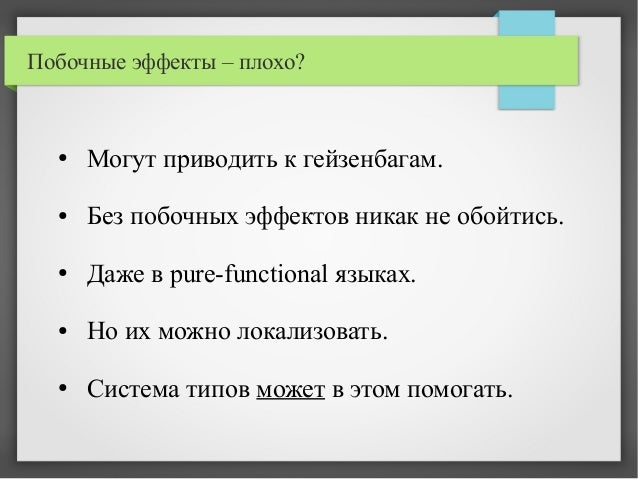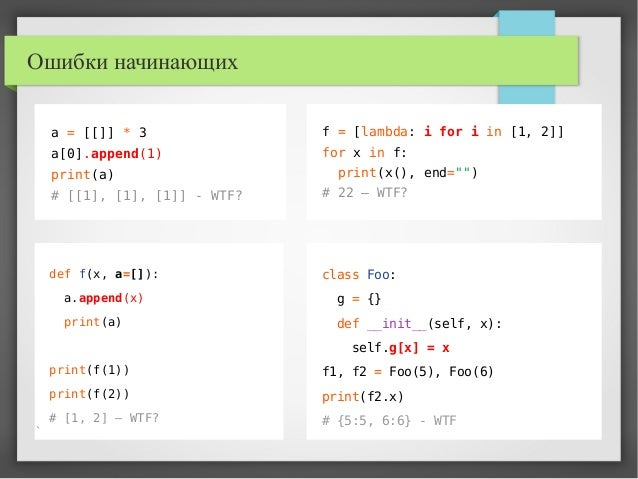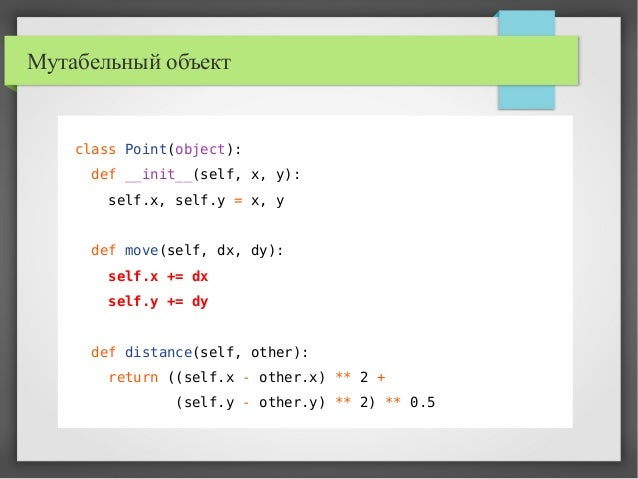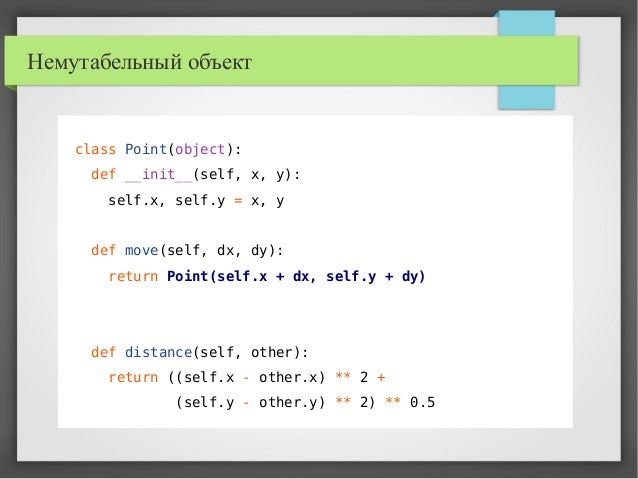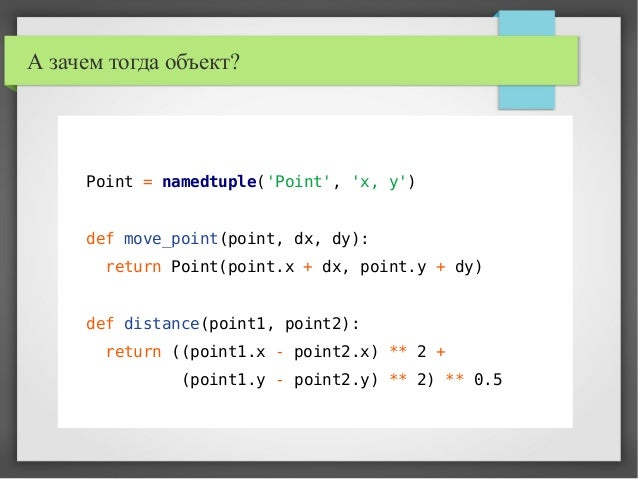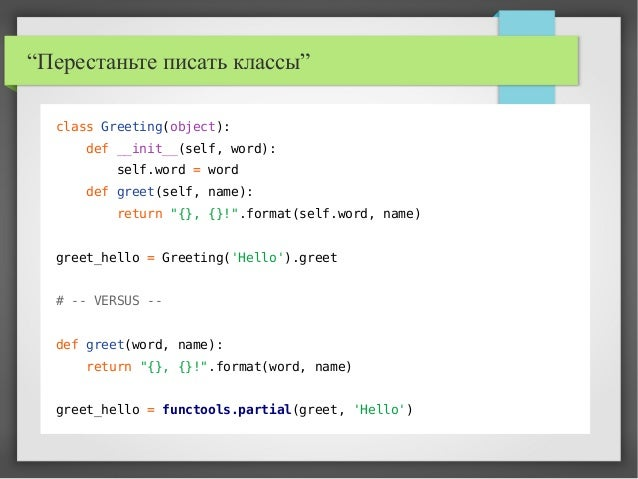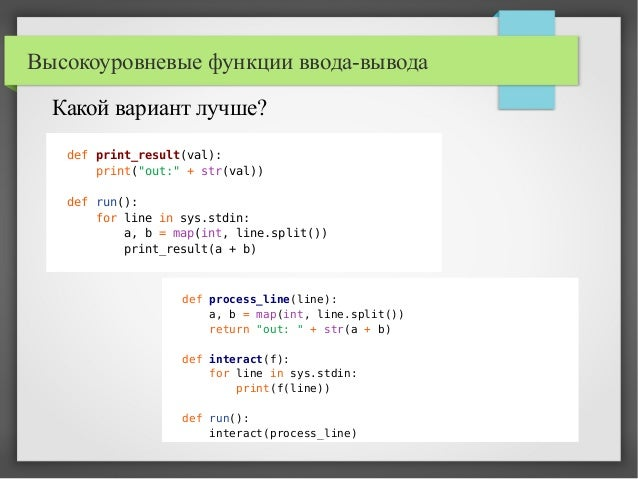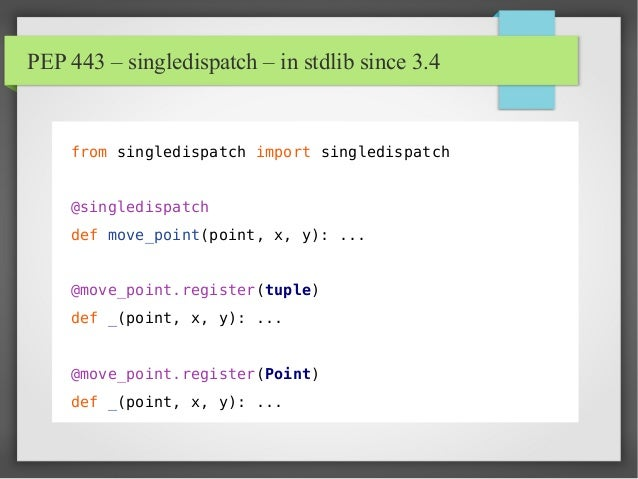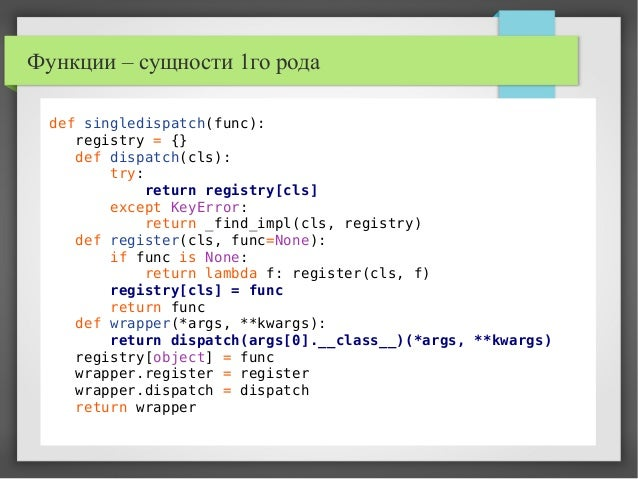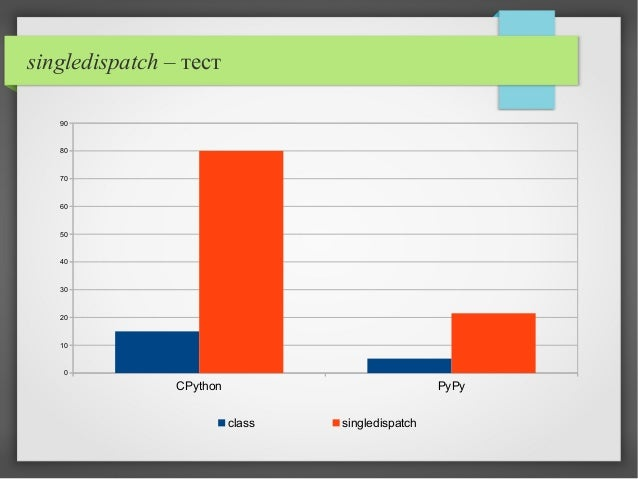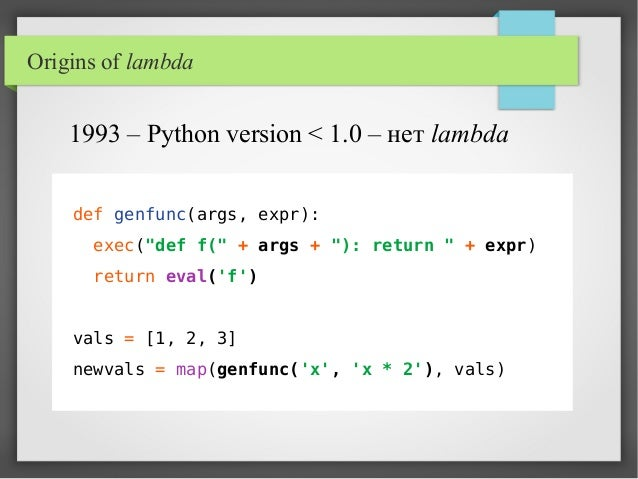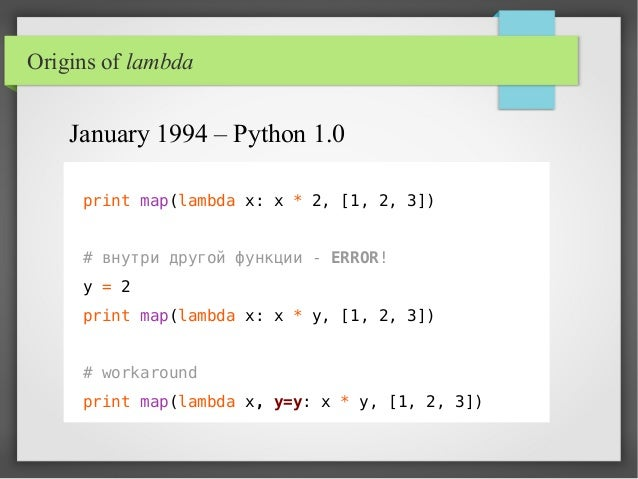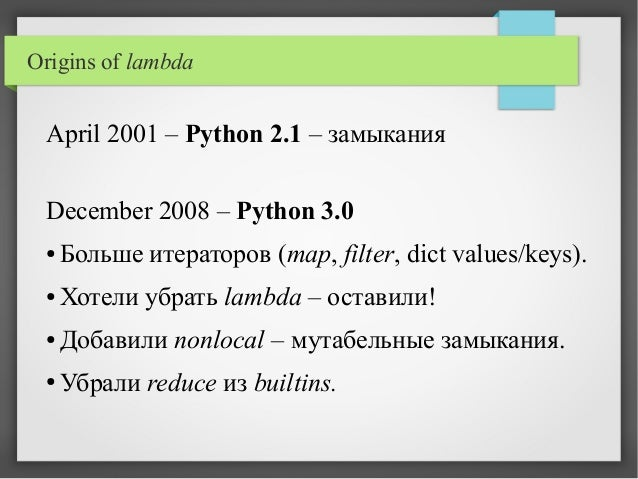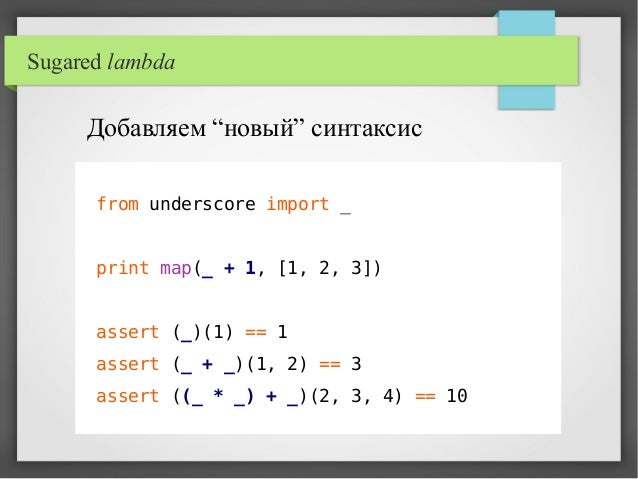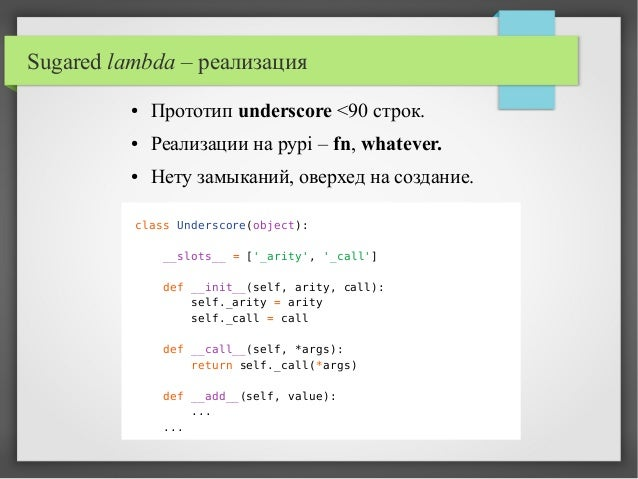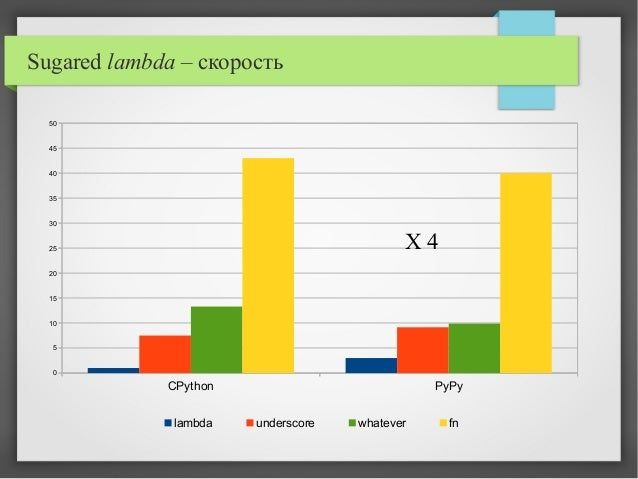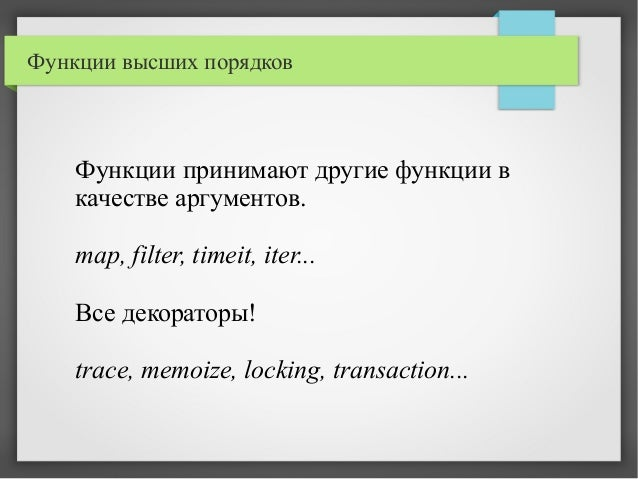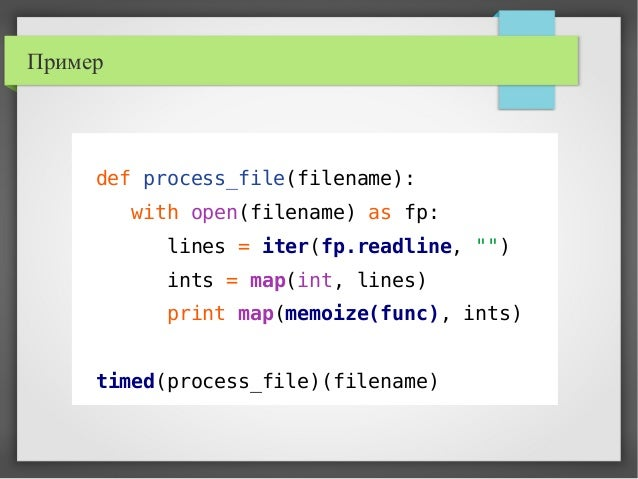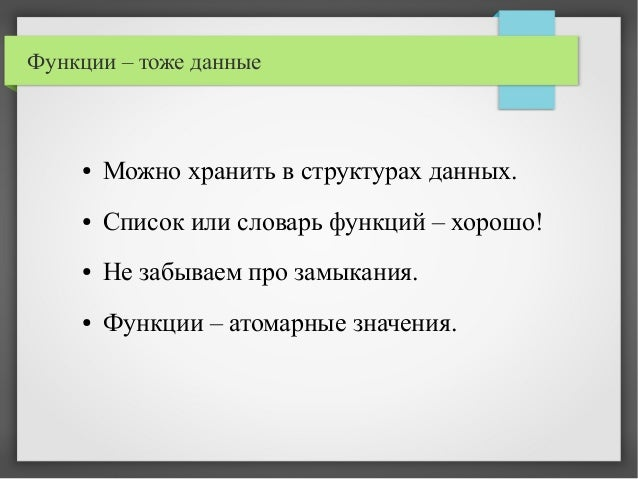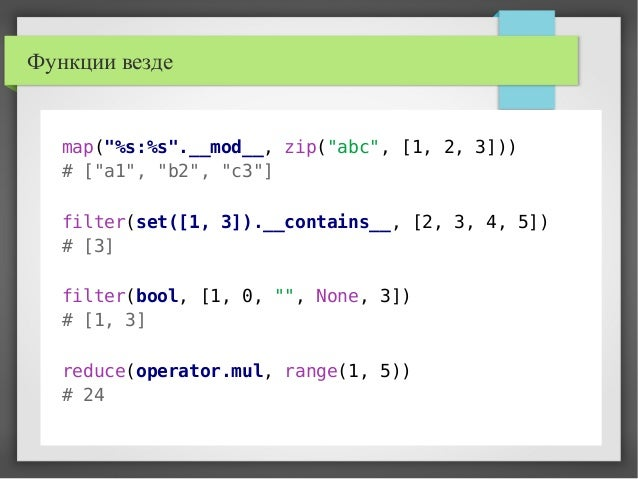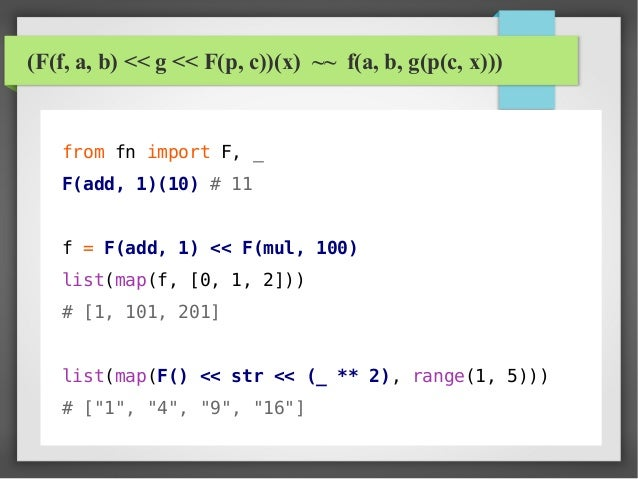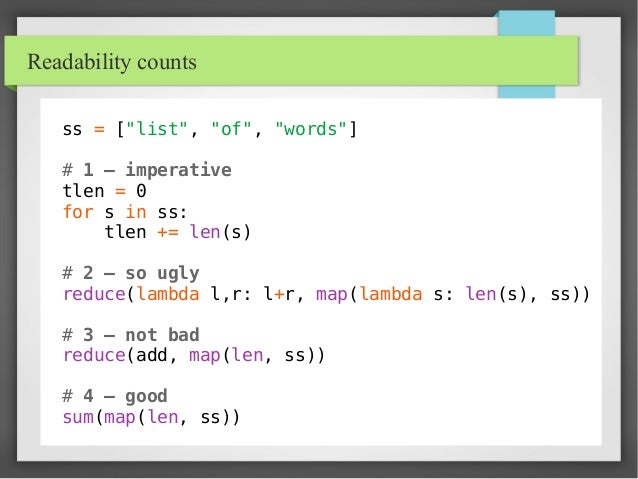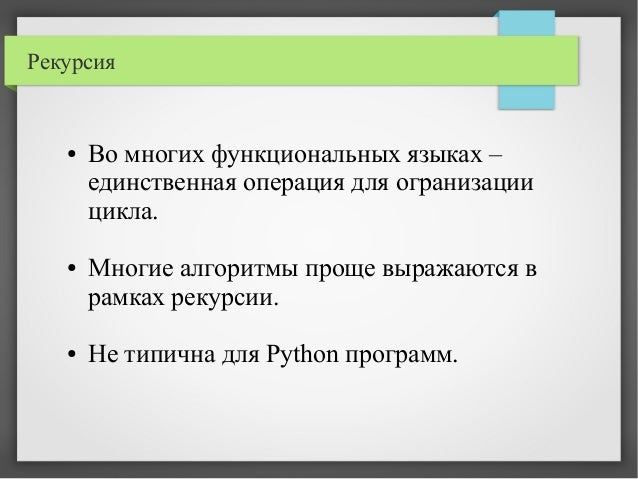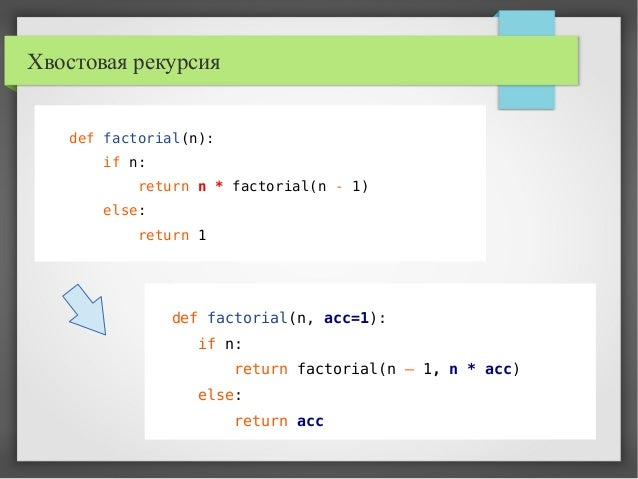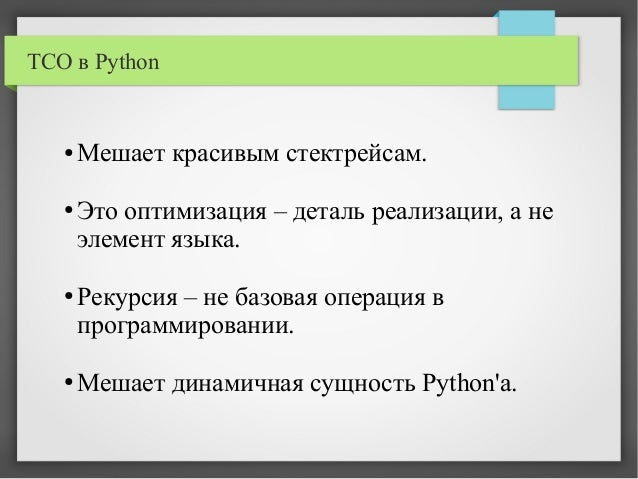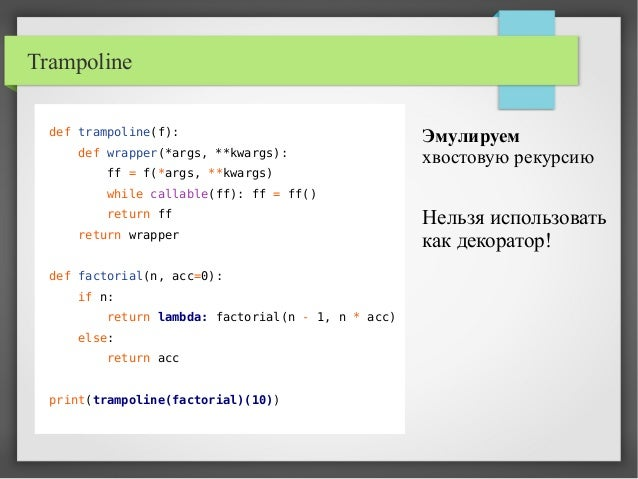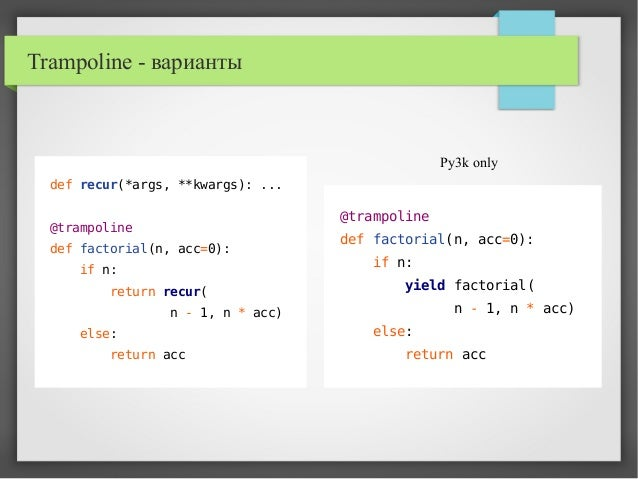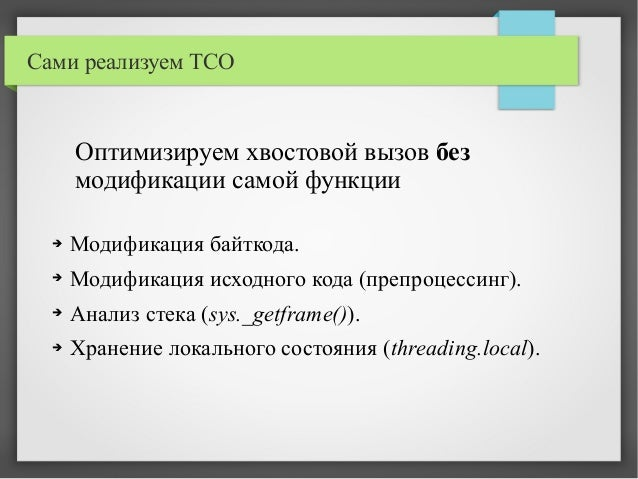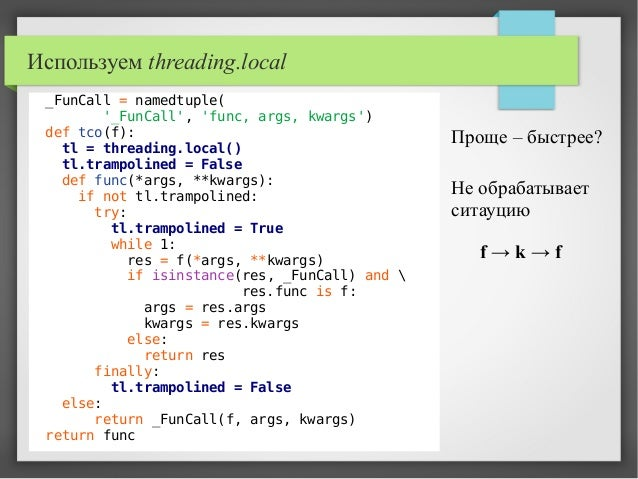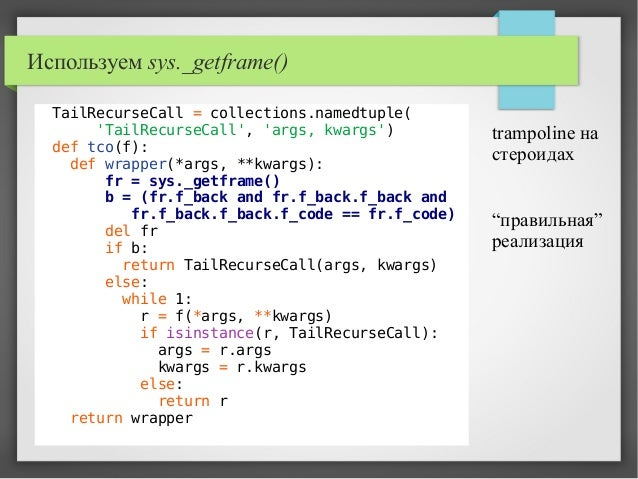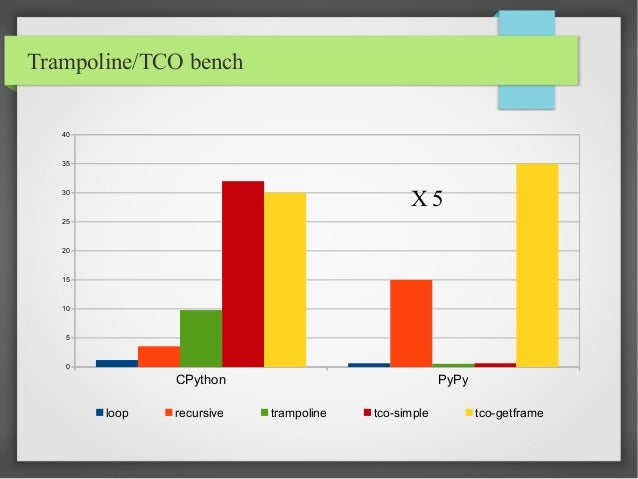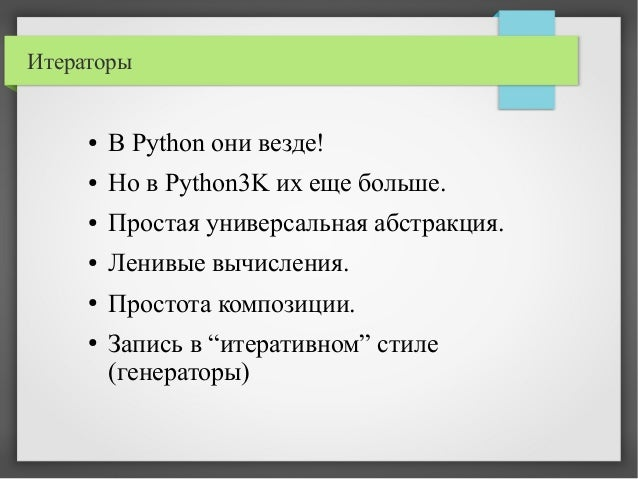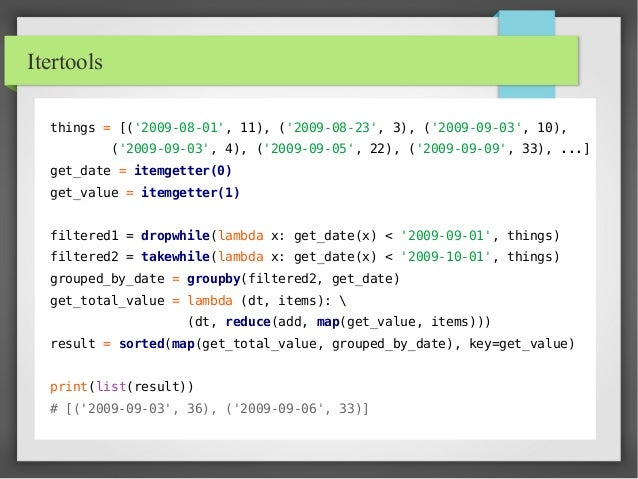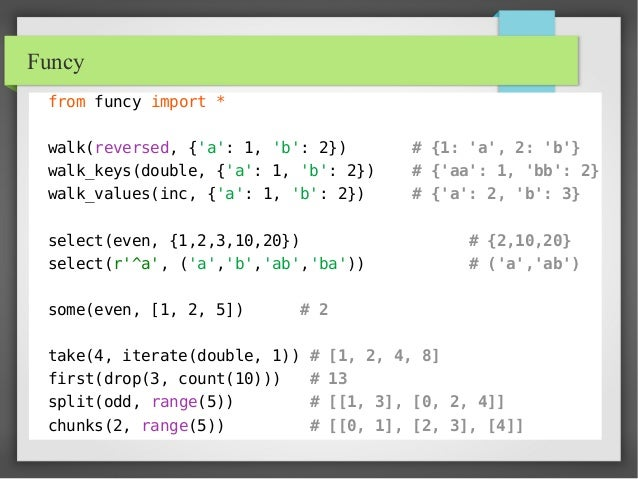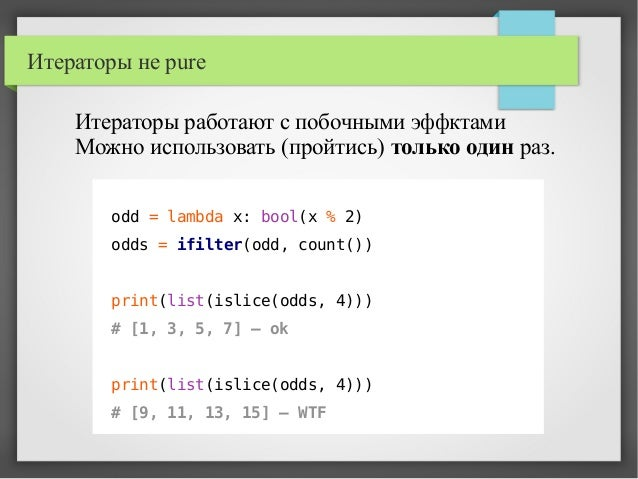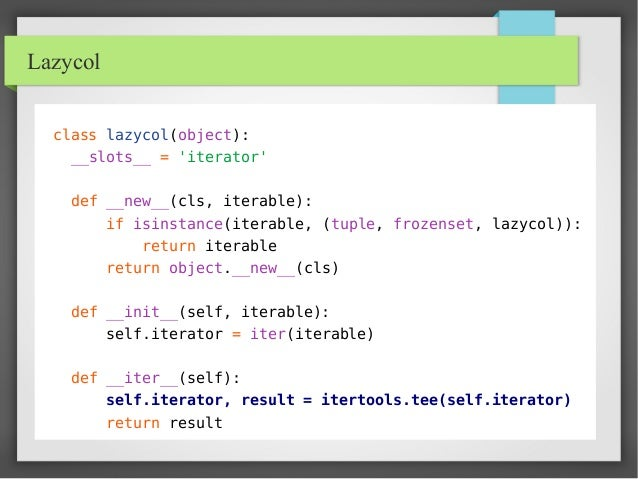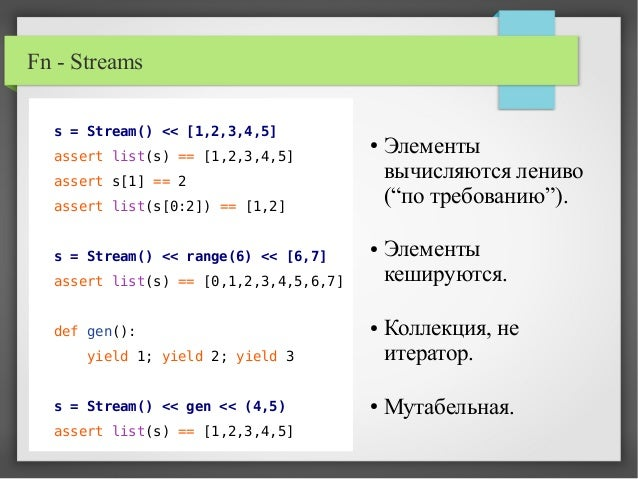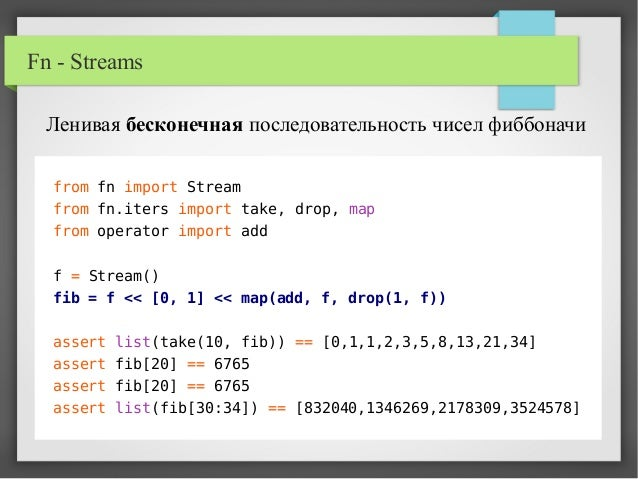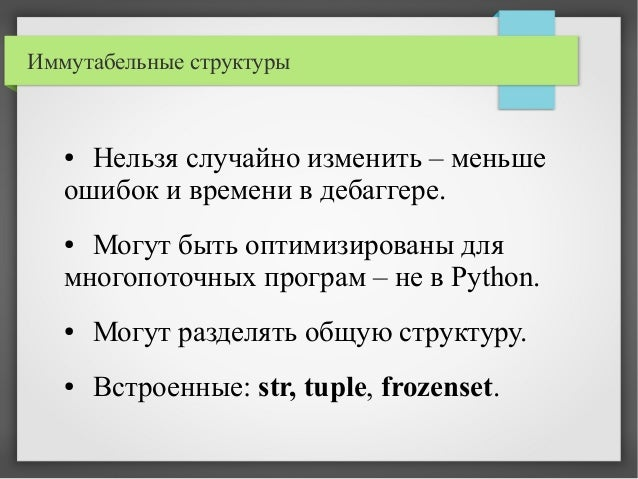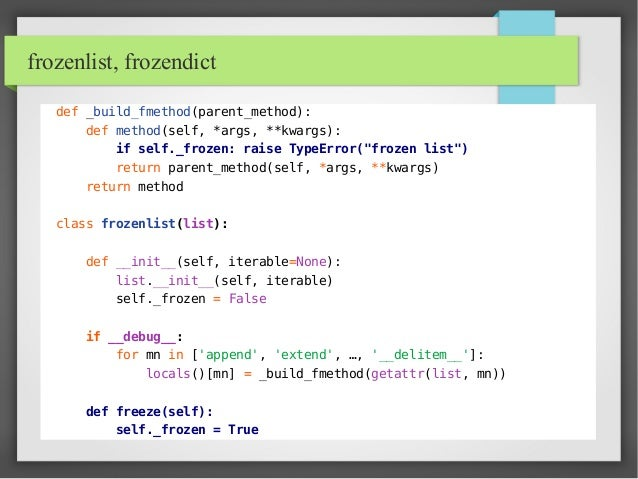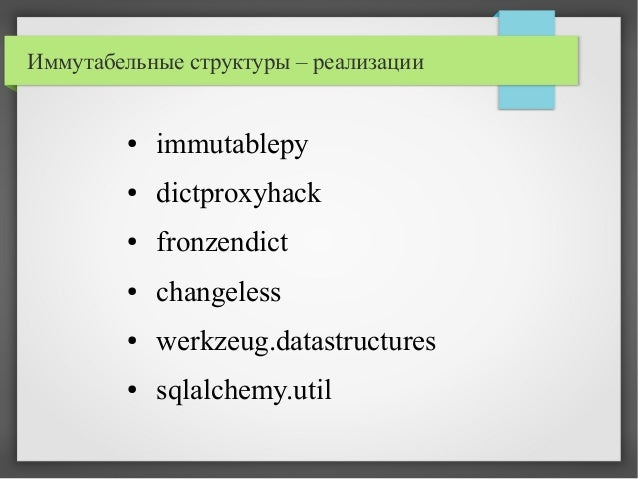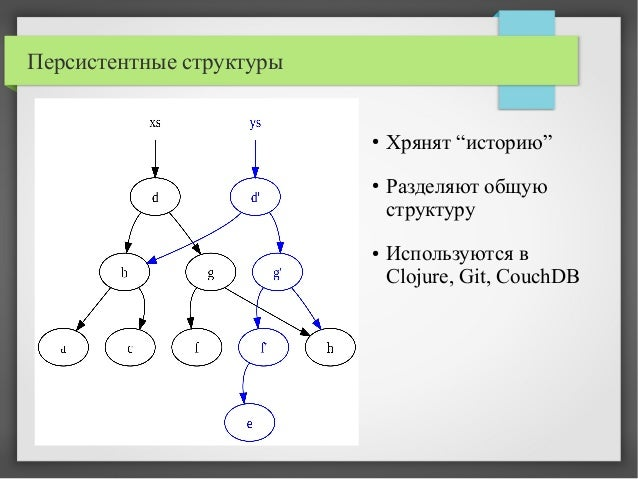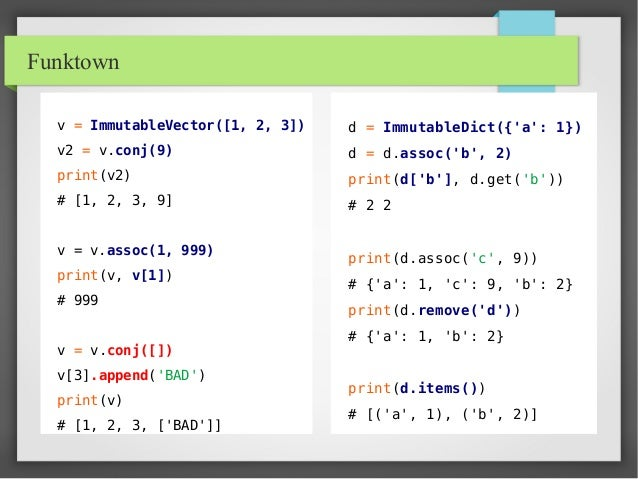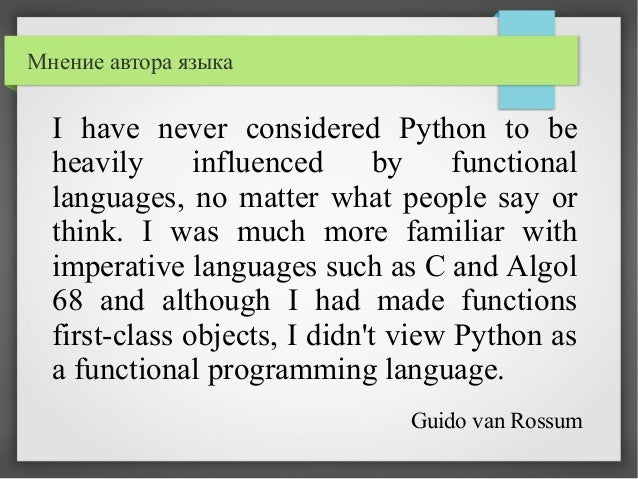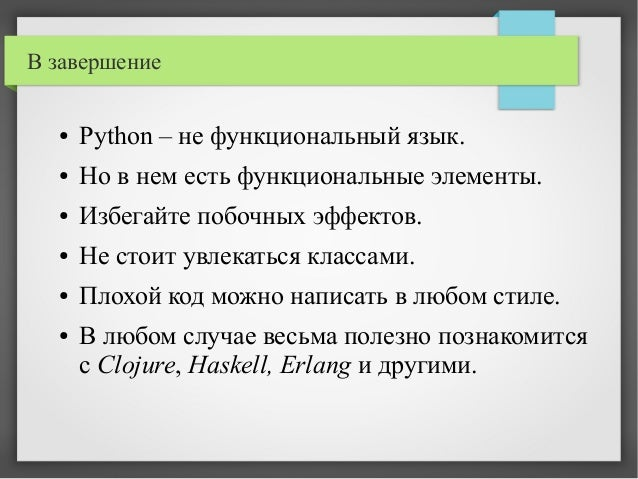Upcoming SlideShare
×
• Full Name
Comment goes here.

Are you sure you want to Yes No• Be the first to comment

### Pyton – пробуем функциональный стиль

1. 1. Pyton – пробуем функциональный стиль Жлобич Андрей Wargaming.net Python Developer Minsk Python Meetup
2. 2. Принципы ФП ✔ Чистота – нет побочных эффектов. ✔ Функции – сущности 1го рода. ✔ Функции высших порядков. ✔ Замыкания. ✔ Рекурсия. ✔ Неизменяемые структуры. ✔ Ленивые вычисления.
3. 3. Versus ➔ Императивный стиль ➔ Функциональный Декларативный стиль grep 'search-for' inputfile.txt > s1.txt sort s1.txt > s2.txt uniq s2.txt > result.txt rm s1.txt s2.txt cat inputfile.txt | grep 'search-for' | sort | uniq | cat > result.txt
4. 4. Чистые функции def p_fact(n): # pure return 1 if n < 2 else n * p_fact(n - 1) def c_fact(n): # referentially transparent try: return c_fact._cache[n] except KeyError: x = c_fact._cache[n] = p_fact(n) return x c_fact._cache = {} def o_fact(n): # io + logic - bad f = p_fact(n) print("{}! = {}".format(n, f) return f calculated_factorials = {} def g_fact(n): # side effents f = fact(n) calculated_factorials[n] = f return f
5. 5. Побочные эффекты – плохо? ● Могут приводить к гейзенбагам. ● Без побочных эффектов никак не обойтись. ● Даже в pure-functional языках. ● Но их можно локализовать. ● Система типов может в этом помогать.
6. 6. Ошибки начинающих a = [[]] * 3 a.append(1) print(a) # [, , ] - WTF? class Foo: g = {} def __init__(self, x): self.g[x] = x f1, f2 = Foo(5), Foo(6) print(f2.x) # {5:5, 6:6} - WTF f = [lambda: i for i in [1, 2]] for x in f: print(x(), end="") # 22 – WTF? def f(x, a=[]): a.append(x) print(a) print(f(1)) print(f(2)) # [1, 2] – WTF? `
7. 7. Мутабельный объект class Point(object): def __init__(self, x, y): self.x, self.y = x, y def move(self, dx, dy): self.x += dx self.y += dy def distance(self, other): return ((self.x - other.x) ** 2 + (self.y - other.y) ** 2) ** 0.5
8. 8. Немутабельный объект class Point(object): def __init__(self, x, y): self.x, self.y = x, y def move(self, dx, dy): return Point(self.x + dx, self.y + dy) def distance(self, other): return ((self.x - other.x) ** 2 + (self.y - other.y) ** 2) ** 0.5
9. 9. А зачем тогда объект? Point = namedtuple('Point', 'x, y') def move_point(point, dx, dy): return Point(point.x + dx, point.y + dy) def distance(point1, point2): return ((point1.x - point2.x) ** 2 + (point1.y - point2.y) ** 2) ** 0.5
10. 10. “Перестаньте писать классы” class Greeting(object): def __init__(self, word): self.word = word def greet(self, name): return "{}, {}!".format(self.word, name) greet_hello = Greeting('Hello').greet # -- VERSUS -- def greet(word, name): return "{}, {}!".format(word, name) greet_hello = functools.partial(greet, 'Hello')
11. 11. Высокоуровневые функции ввода-вывода def process_line(line): a, b = map(int, line.split()) return "out: " + str(a + b) def interact(f): for line in sys.stdin: print(f(line)) def run(): interact(process_line) Какой вариант лучше? def print_result(val): print("out:" + str(val)) def run(): for line in sys.stdin: a, b = map(int, line.split()) print_result(a + b)
12. 12. PEP 443 – singledispatch – in stdlib since 3.4 from singledispatch import singledispatch @singledispatch def move_point(point, x, y): ... @move_point.register(tuple) def _(point, x, y): ... @move_point.register(Point) def _(point, x, y): ...
13. 13. Функции – сущности 1го рода def singledispatch(func): registry = {} def dispatch(cls): try: return registry[cls] except KeyError: return _find_impl(cls, registry) def register(cls, func=None): if func is None: return lambda f: register(cls, f) registry[cls] = func return func def wrapper(*args, **kwargs): return dispatch(args.__class__)(*args, **kwargs) registry[object] = func wrapper.register = register wrapper.dispatch = dispatch return wrapper
14. 14. singledispatch – тест CPython PyPy 0 10 20 30 40 50 60 70 80 90 class singledispatch
15. 15. Origins of lambda def genfunc(args, expr): exec("def f(" + args + "): return " + expr) return eval('f') vals = [1, 2, 3] newvals = map(genfunc('x', 'x * 2'), vals) 1993 – Python version < 1.0 – нет lambda
16. 16. print map(lambda x: x * 2, [1, 2, 3]) # внутри другой функции - ERROR! y = 2 print map(lambda x: x * y, [1, 2, 3]) # workaround print map(lambda x, y=y: x * y, [1, 2, 3]) January 1994 – Python 1.0 Origins of lambda
17. 17. Origins of lambda April 2001 – Python 2.1 – замыкания December 2008 – Python 3.0 ● Больше итераторов (map, filter, dict values/keys). ● Хотели убрать lambda – оставили! ● Добавили nonlocal – мутабельные замыкания. ● Убрали reduce из builtins.
18. 18. Sugared lambda Добавляем “новый” синтаксис from underscore import _ print map(_ + 1, [1, 2, 3]) assert (_)(1) == 1 assert (_ + _)(1, 2) == 3 assert ((_ * _) + _)(2, 3, 4) == 10
19. 19. Sugared lambda – реализация ● Прототип underscore <90 строк. ● Реализации на pypi – fn, whatever. ● Нету замыканий, оверхед на создание. class Underscore(object): __slots__ = ['_arity', '_call'] def __init__(self, arity, call): self._arity = arity self._call = call def __call__(self, *args): return self._call(*args) def __add__(self, value): ... ...
20. 20. Sugared lambda – скорость CPython PyPy 0 5 10 15 20 25 30 35 40 45 50 lambda underscore whatever fn X 4
21. 21. Функции высших порядков Функции принимают другие функции в качестве аргументов. map, filter, timeit, iter... Все декораторы! trace, memoize, locking, transaction...
22. 22. Пример def process_file(filename): with open(filename) as fp: lines = iter(fp.readline, "") ints = map(int, lines) print map(memoize(func), ints) timed(process_file)(filename)
23. 23. Функции – тоже данные ● Можно хранить в структурах данных. ● Список или словарь функций – хорошо! ● Не забываем про замыкания. ● Функции – атомарные значения.
24. 24. Функции везде map("%s:%s".__mod__, zip("abc", [1, 2, 3])) # ["a1", "b2", "c3"] filter(set([1, 3]).__contains__, [2, 3, 4, 5]) #  filter(bool, [1, 0, "", None, 3]) # [1, 3] reduce(operator.mul, range(1, 5)) # 24
25. 25. (F(f, a, b) << g << F(p, c))(x) ~~ f(a, b, g(p(c, x))) from fn import F, _ F(add, 1)(10) # 11 f = F(add, 1) << F(mul, 100) list(map(f, [0, 1, 2])) # [1, 101, 201] list(map(F() << str << (_ ** 2), range(1, 5))) # ["1", "4", "9", "16"]
26. 26. Readability counts ss = ["list", "of", "words"] # 1 – imperative tlen = 0 for s in ss: tlen += len(s) # 2 – so ugly reduce(lambda l,r: l+r, map(lambda s: len(s), ss)) # 3 – not bad reduce(add, map(len, ss)) # 4 – good sum(map(len, ss))
27. 27. Рекурсия ● Во многих функциональных языках – единственная операция для огранизации цикла. ● Многие алгоритмы проще выражаются в рамках рекурсии. ● Не типична для Python программ.
28. 28. Хвостовая рекурсия def factorial(n): if n: return n * factorial(n - 1) else: return 1 def factorial(n, acc=1): if n: return factorial(n – 1, n * acc) else: return acc
29. 29. TCO в Python ● Мешает красивым стектрейсам. ● Это оптимизация – деталь реализации, а не элемент языка. ● Рекурсия – не базовая операция в программировании. ● Мешает динамичная сущность Python'а.
30. 30. Trampoline def trampoline(f): def wrapper(*args, **kwargs): ff = f(*args, **kwargs) while callable(ff): ff = ff() return ff return wrapper def factorial(n, acc=0): if n: return lambda: factorial(n - 1, n * acc) else: return acc print(trampoline(factorial)(10)) Эмулируем хвостовую рекурсию Нельзя использовать как декоратор!
31. 31. Trampoline - варианты def recur(*args, **kwargs): ... @trampoline def factorial(n, acc=0): if n: return recur( n - 1, n * acc) else: return acc @trampoline def factorial(n, acc=0): if n: yield factorial( n - 1, n * acc) else: return acc Py3k only
32. 32. Сами реализуем TCO ➔ Модификация байткода. ➔ Модификация исходного кода (препроцессинг). ➔ Анализ стека (sys._getframe()). ➔ Хранение локального состояния (threading.local). Оптимизируем хвостовой вызов без модификации самой функции
33. 33. Используем threading.local _FunCall = namedtuple( '_FunCall', 'func, args, kwargs') def tco(f): tl = threading.local() tl.trampolined = False def func(*args, **kwargs): if not tl.trampolined: try: tl.trampolined = True while 1: res = f(*args, **kwargs) if isinstance(res, _FunCall) and res.func is f: args = res.args kwargs = res.kwargs else: return res finally: tl.trampolined = False else: return _FunCall(f, args, kwargs) return func Проще – быстрее? Не обрабатывает ситауцию f → k → f
34. 34. Используем sys._getframe() TailRecurseCall = collections.namedtuple( 'TailRecurseCall', 'args, kwargs') def tco(f): def wrapper(*args, **kwargs): fr = sys._getframe() b = (fr.f_back and fr.f_back.f_back and fr.f_back.f_back.f_code == fr.f_code) del fr if b: return TailRecurseCall(args, kwargs) else: while 1: r = f(*args, **kwargs) if isinstance(r, TailRecurseCall): args = r.args kwargs = r.kwargs else: return r return wrapper trampoline на стероидах “правильная” реализация
35. 35. Trampoline/TCO bench CPython PyPy 0 5 10 15 20 25 30 35 40 loop recursive trampoline tco-simple tco-getframe X 5
36. 36. Итераторы ● В Python они везде! ● Но в Python3K их еще больше. ● Простая универсальная абстракция. ● Ленивые вычисления. ● Простота композиции. ● Запись в “итеративном” стиле (генераторы)
37. 37. Itertools things = [('2009-08-01', 11), ('2009-08-23', 3), ('2009-09-03', 10), ('2009-09-03', 4), ('2009-09-05', 22), ('2009-09-09', 33), ...] get_date = itemgetter(0) get_value = itemgetter(1) filtered1 = dropwhile(lambda x: get_date(x) < '2009-09-01', things) filtered2 = takewhile(lambda x: get_date(x) < '2009-10-01', things) grouped_by_date = groupby(filtered2, get_date) get_total_value = lambda (dt, items): (dt, reduce(add, map(get_value, items))) result = sorted(map(get_total_value, grouped_by_date), key=get_value) print(list(result)) # [('2009-09-03', 36), ('2009-09-06', 33)]
38. 38. Funcy from funcy import * walk(reversed, {'a': 1, 'b': 2}) # {1: 'a', 2: 'b'} walk_keys(double, {'a': 1, 'b': 2}) # {'aa': 1, 'bb': 2} walk_values(inc, {'a': 1, 'b': 2}) # {'a': 2, 'b': 3} select(even, {1,2,3,10,20}) # {2,10,20} select(r'^a', ('a','b','ab','ba')) # ('a','ab') some(even, [1, 2, 5]) # 2 take(4, iterate(double, 1)) # [1, 2, 4, 8] first(drop(3, count(10))) # 13 split(odd, range(5)) # [[1, 3], [0, 2, 4]] chunks(2, range(5)) # [[0, 1], [2, 3], ]
39. 39. Итераторы не pure odd = lambda x: bool(x % 2) odds = ifilter(odd, count()) print(list(islice(odds, 4))) # [1, 3, 5, 7] – ok print(list(islice(odds, 4))) # [9, 11, 13, 15] – WTF Итераторы работают с побочными эффктами Можно использовать (пройтись) только один раз.
40. 40. Lazycol class lazycol(object): __slots__ = 'iterator' def __new__(cls, iterable): if isinstance(iterable, (tuple, frozenset, lazycol)): return iterable return object.__new__(cls) def __init__(self, iterable): self.iterator = iter(iterable) def __iter__(self): self.iterator, result = itertools.tee(self.iterator) return result
41. 41. Fn - Streams s = Stream() << [1,2,3,4,5] assert list(s) == [1,2,3,4,5] assert s == 2 assert list(s[0:2]) == [1,2] s = Stream() << range(6) << [6,7] assert list(s) == [0,1,2,3,4,5,6,7] def gen(): yield 1; yield 2; yield 3 s = Stream() << gen << (4,5) assert list(s) == [1,2,3,4,5] ● Элементы вычисляются лениво (“по требованию”). ● Элементы кешируются. ● Коллекция, не итератор. ● Мутабельная.
42. 42. Fn - Streams from fn import Stream from fn.iters import take, drop, map from operator import add f = Stream() fib = f << [0, 1] << map(add, f, drop(1, f)) assert list(take(10, fib)) == [0,1,1,2,3,5,8,13,21,34] assert fib == 6765 assert fib == 6765 assert list(fib[30:34]) == [832040,1346269,2178309,3524578] Ленивая бесконечная последовательность чисел фиббоначи
43. 43. Иммутабельные структуры ● Нельзя случайно изменить – меньше ошибок и времени в дебаггере. ● Могут быть оптимизированы для многопоточных програм – не в Python. ● Могут разделять общую структуру. ● Встроенные: str, tuple, frozenset.
44. 44. frozenlist, frozendict def _build_fmethod(parent_method): def method(self, *args, **kwargs): if self._frozen: raise TypeError("frozen list") return parent_method(self, *args, **kwargs) return method class frozenlist(list): def __init__(self, iterable=None): list.__init__(self, iterable) self._frozen = False if __debug__: for mn in ['append', 'extend', …, '__delitem__']: locals()[mn] = _build_fmethod(getattr(list, mn)) def freeze(self): self._frozen = True
45. 45. Иммутабельные структуры – реализации ● immutablepy ● dictproxyhack ● fronzendict ● changeless ● werkzeug.datastructures ● sqlalchemy.util
46. 46. Персистентные структуры ● Хрянят “историю” ● Разделяют общую структуру ● Используются в Clojure, Git, CouchDB
47. 47. Funktown v = ImmutableVector([1, 2, 3]) v2 = v.conj(9) print(v2) # [1, 2, 3, 9] v = v.assoc(1, 999) print(v, v) # 999 v = v.conj([]) v.append('BAD') print(v) # [1, 2, 3, ['BAD']] d = ImmutableDict({'a': 1}) d = d.assoc('b', 2) print(d['b'], d.get('b')) # 2 2 print(d.assoc('c', 9)) # {'a': 1, 'c': 9, 'b': 2} print(d.remove('d')) # {'a': 1, 'b': 2} print(d.items()) # [('a', 1), ('b', 2)]
48. 48. I have never considered Python to be heavily influenced by functional languages, no matter what people say or think. I was much more familiar with imperative languages such as C and Algol 68 and although I had made functions first-class objects, I didn't view Python as a functional programming language. Мнение автора языка Guido van Rossum
49. 49. В завершение ● Python – не функциональный язык. ● Но в нем есть функциональные элементы. ● Избегайте побочных эффектов. ● Не стоит увлекаться классами. ● Плохой код можно написать в любом стиле. ● В любом случае весьма полезно познакомится с Clojure, Haskell, Erlang и другими.
50. 50. Minsk Python Meetup Всем большое спасибо за внимание вопросы? a.zhlobich@gmail.com anjensan@jabber.ru anjensan at github, habrahabr etc